﻿ 基于多项式的二维PSD非线性校正算法研究
 舰船科学技术2019, Vol. 41Issue (6): 89-93PDF

Research on nonlinear correction algorithm of two-dimensional psd based on polynomials
ZHANG Ji-ming
The 713 Research Institute of CSIC, Zhengzhou 450001, China
Abstract: Aiming at the nonlinearity of position sensitive detector (PSD), the existing nonlinear correction methods are analyzed synthetically, and a polynomial fitting algorithm is proposed to correct the nonlinearity of PSD. Two dimensional PSD calibration system is built using two-dimensional translation table, and polynomial correction method is discussed in detail. By comparing the experimental data, the binary cubic polynomial is selected to complete the correction. The correction polynomial correction algorithm is verified by the measurement of different points on the PSD surface. The experimental results show that the mean deviation of the corrected measurement error is less than 0.0185.
Key words: PSD     nonlinearity correction     polynomial fitting
0 引　言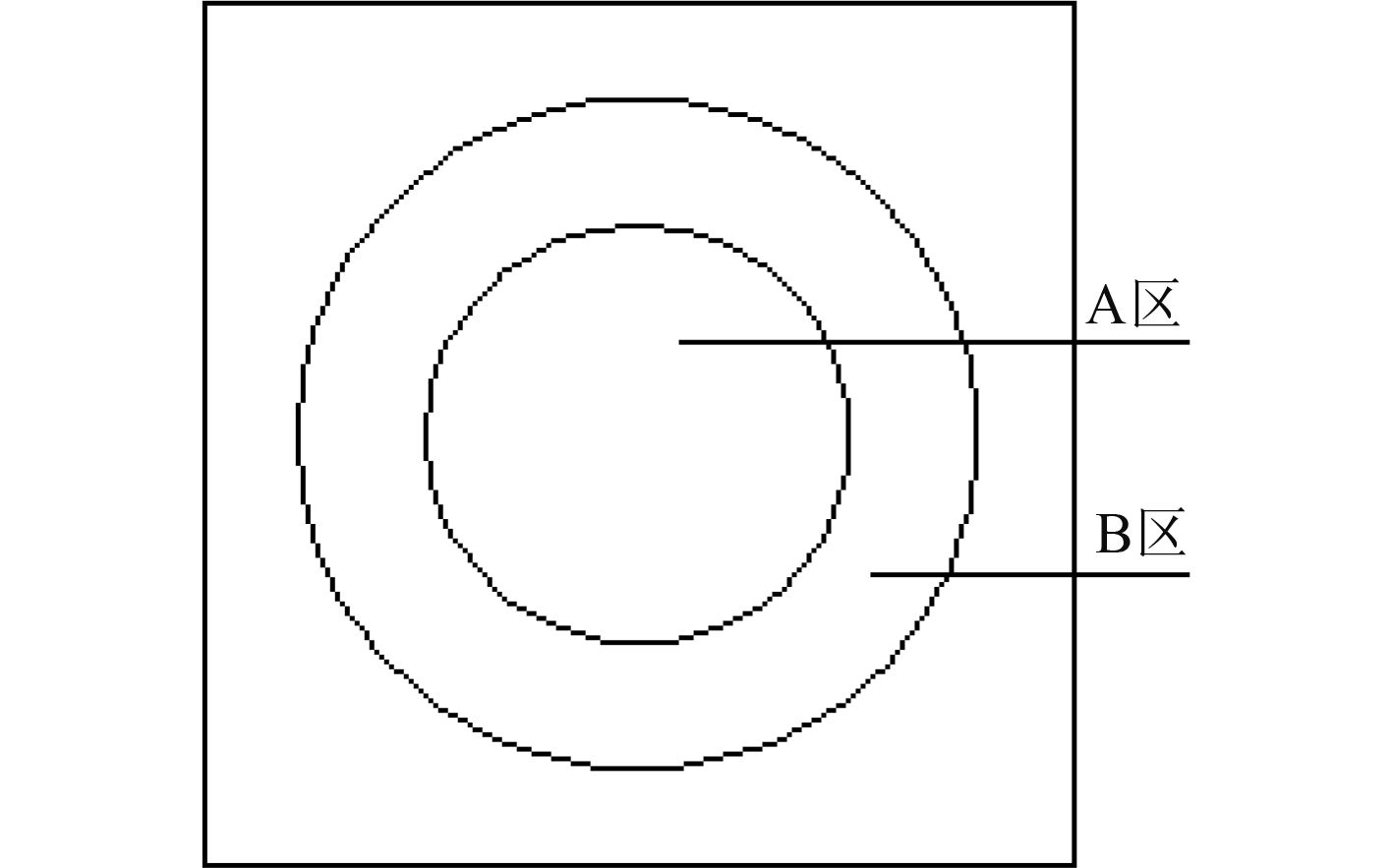图 1 PSD的A区和B区 Fig. 1 Regions A and B of PSD

1 多项式校正非线性算法

 $\Delta x = {E_x}({x_i},{y_j}) = {x_i} - {x_{i0}}\text{，}$ (1)
 $\Delta y = {E_y}({x_i},{y_j}) = {y_j} - {y_{j0}}\text{。}$ (2)

 ${{E}_x}({x_i},{y_j}) = a{x^2} + b{y^2} + cxy + dx + ey + f\text{，}$ (3)
 ${{E}_y}({x_i},{y_j}) = {a_1}{x^2} + {b_1}{y^2} + {c_1}xy + {d_1}x + {e_1}y + {f_1}\text{；}$ (4)

 $\begin{split} {{E}_x}({x_i},{y_j}) = & a{x^3} + b{y^3} + c{x^2}y + dx{y^2} + e{x^2} +\\ & f{y^2} + gxy + hx + iy + k\text{，} \end{split}$ (5)
 $\begin{split} {{E}_y}({x_i},{y_j}) =& {a_1}{x^3} + {b_1}{y^3} + {c_1}{x^2}y + {d_1}x{y^2} +\\ & {e_1}{x^2} + {f_1}{y^2} + {g_1}xy + {h_1}x + {i_1}y + {k_1}\text{；} \end{split}$ (6)

 $\begin{split} {{E}_x}({x_i},{y_j}) = a{x^4} + b{y^4} + c{x^3}y + dx{y^3} + e{x^2}{y^2} + f{x^3} + \\ g{y^3} +h{x^2}y + ix{y^2} + j{x^2} + k{y^2} + lxy + mx + ny + p \text{，} \end{split}$ (7)
 $\begin{split} {{E}_y}({x_i},{y_j}) =& {a_1}{x^4} + {b_1}{y^4} + {c_1}{x^3}y + {d_1}x{y^3} + {e_1}{x^2}{y^2} + {f_1}{x^3} + \\ &{g_1}{y^3} + {h_1}{x^2}y + {i_1}x{y^2} + {j_1}{x^2} + {k_1}{y^2} + {l_1}xy +\\ &{m_1}x + {n_1}y + {k_1} \text{。} \end{split}$ (8)

 $\scriptsize A = \left( {\begin{array}{*{20}{c}} {x_1^3} \!\!\!\!&\!\!\!\! {y_1^3} \!\!\!\!&\!\!\!\! {x_1^2{y_1}} \!\!\!\!&\!\!\!\! {{x_1}y_1^2} \!\!\!\!&\!\!\!\! {x_1^2} \!\!\!\!&\!\!\!\! {y_1^2} \!\!\!\!&\!\!\!\! {{x_1}{y_1}} \!\!\!\!&\!\!\!\! {{x_1}} \!\!\!\!&\!\!\!\! {{y_1}} \!\!\!\!&\!\!\!\! 1 \\ {x_2^3} \!\!\!\!&\!\!\!\! {y_2^3} \!\!\!\!&\!\!\!\! {x_2^2{y_2}} \!\!\!\!&\!\!\!\! {{x_2}y_2^2} \!\!\!\!&\!\!\!\! {x_2^2} \!\!\!\!&\!\!\!\! {y_2^2} \!\!\!\!&\!\!\!\! {{x_2}{y_2}} \!\!\!\!&\!\!\!\! {{x_2}} \!\!\!\!&\!\!\!\! {{y_2}} \!\!\!\!&\!\!\!\! 1 \\ {x_3^3} \!\!\!\!&\!\!\!\! {y_3^3} \!\!\!\!&\!\!\!\! {x_3^2{y_3}} \!\!\!\!&\!\!\!\! {{x_3}y_3^2} \!\!\!\!&\!\!\!\! {x_3^2} \!\!\!\!&\!\!\!\! {y_3^2} \!\!\!\!&\!\!\!\! {{x_3}{y_3}} \!\!\!\!&\!\!\!\! {{x_3}} \!\!\!\!&\!\!\!\! {{y_3}} \!\!\!\!&\!\!\!\! 1 \\ {x_4^3} \!\!\!\!&\!\!\!\! {y_4^3} \!\!\!\!&\!\!\!\! {x_4^2{y_4}} \!\!\!\!&\!\!\!\! {{x_4}y_4^2} \!\!\!\!&\!\!\!\! {x_4^2} \!\!\!\!&\!\!\!\! {y_4^2} \!\!\!\!&\!\!\!\! {{x_4}{y_4}} \!\!\!\!&\!\!\!\! {{x_4}} \!\!\!\!&\!\!\!\! {{y_4}} \!\!\!\!&\!\!\!\! 1 \\ \vdots \!\!\!\!&\!\!\!\! \vdots \!\!\!\!&\!\!\!\! \vdots \!\!\!\!&\!\!\!\! \vdots \!\!\!\!&\!\!\!\! \vdots \!\!\!\!&\!\!\!\! \vdots \!\!\!\!&\!\!\!\! \vdots \!\!\!\!&\!\!\!\! \vdots \!\!\!\!&\!\!\!\! \vdots \!\!\!\!&\!\!\!\! \vdots \\ {x_{n - 1}^3} \!\!\!\!&\!\!\!\! {y_{n - 1}^3} \!\!\!\!&\!\!\!\! {x_{n - 1}^2{y_{n - 1}}} \!\!\!\!&\!\!\!\! {{x_{n - 1}}y_{n - 1}^2} \!\!\!\!&\!\!\!\! {x_{n - 1}^2} \!\!\!\!&\!\!\!\! {y_{n - 1}^2} \!\!\!\!&\!\!\!\! {{x_{n - 1}}{y_{n - 1}}} \!\!\!\!&\!\!\!\! {{x_{n - 1}}} \!\!\!\!&\!\!\!\! {{y_{n - 1}}} \!\!\!\!&\!\!\!\! 1 \\ {x_n^3} \!\!\!\!&\!\!\!\! {y_n^3} \!\!\!\!&\!\!\!\! {x_n^2{y_n}} \!\!\!\!&\!\!\!\! {{x_n}y_n^2} \!\!\!\!&\!\!\!\! {x_n^2} \!\!\!\!&\!\!\!\! {y_n^2} \!\!\!\!&\!\!\!\! {{x_n}{y_n}} \!\!\!\!&\!\!\!\! {{x_n}} \!\!\!\!&\!\!\!\! {{y_n}} \!\!\!\!&\!\!\!\! 1 \end{array}} \right)\text{，}$
 ${B = }\left( {\begin{array}{*{20}{c}} {\Delta {x_1}}&{\Delta {y_1}} \\ {\Delta {x_2}}&{\Delta {y_2}} \\ {\Delta {x_3}}&{\Delta {y_3}} \\ {\Delta {x_4}}&{\Delta {y_4}} \\ \vdots &{} \\ {\Delta {x_{n - 1}}}&{\Delta {y_{n - 1}}} \\ {\Delta {x_n}}&{\Delta {y_n}} \end{array}} \right)\text{，} X = \left( {\begin{array}{*{20}{c}} a&{{a_1}} \\ b&{{b_1}} \\ c&{{c_1}} \\ d&{{d_1}} \\ e&{{e_1}} \\ f&{{f_1}} \\ g&g \end{array}} \right)\text{，}$

 ${X = }{\left( {{A^{\rm T}}A} \right)^{ - 1}}{A^{\rm T}}B\text{。}$ (9)

 $B = AX\text{。}$ (10)

 ${X_R} = {X_{out}} - \Delta x\text{，}$ (11)
 ${Y_R} = {Y_{out}} - \Delta y\text{。}$ (12)

1）编写打开文件程序，从文件中读取PSD位置检测装置测量的位置坐标，即读取用来校正的原始数据存储在数组中。

2）根据二维平移台测量的数据与PSD输出的位置坐标，利用式（1）与式（2）分别计算出Δx，Δy

3）利用循环结构计算出每个坐标对应方程的幂次项。

4）利用CMatrix类计算矩阵A与矩阵B，通过矩阵的运算求得矩阵X

5）利用式（11）与式（12）对每一个位置坐标进行修正，求出修正后的均方差，最后把校正后的结果输出到文件中。

2 二维PSD标定系统

2.1 二维平移台选择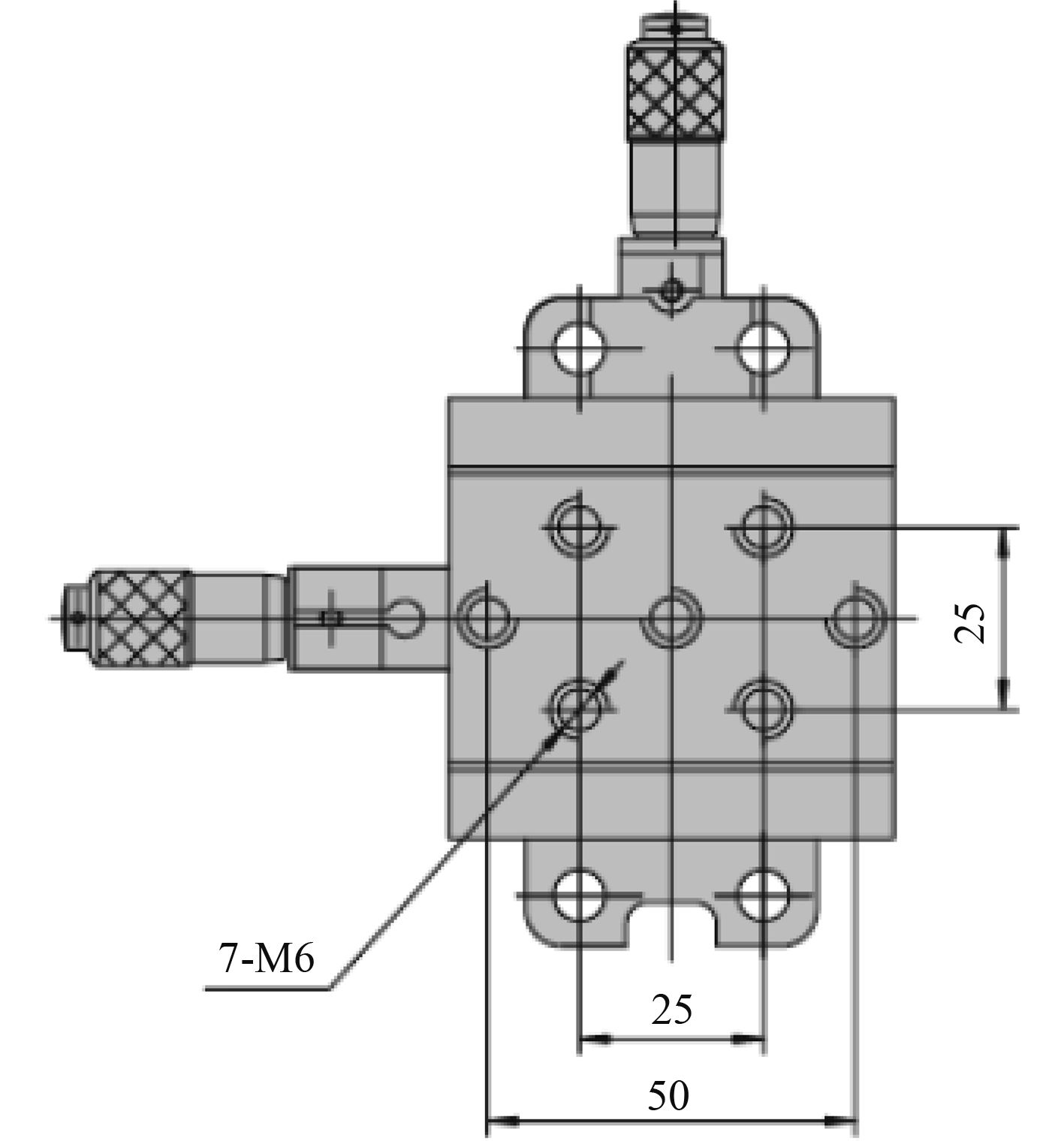图 2 二维平移台结构图 Fig. 2 Structure diagram of two-dimensional translation table
2.2 光源选择

2.3 PSD的选择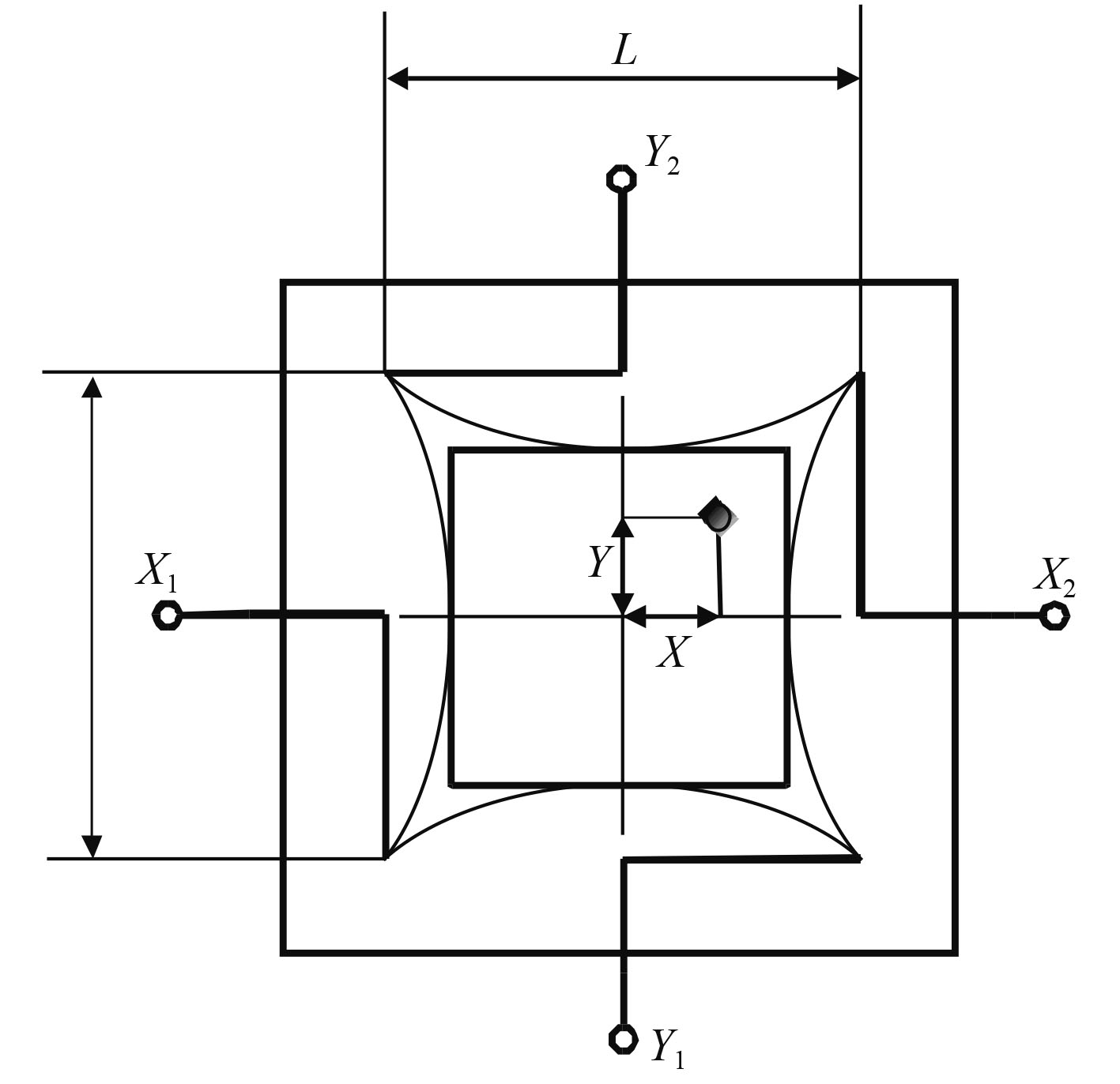图 3 枕型PSD结构 Fig. 3 Occipital PSD structure

PSD-1515具体参数：有效光敏区为15 mm*15 mm，分辨率1 μm，响应时间1 μs，响应光谱范围380 ~1 100 nm，反偏电压为6 V。

3 原始数据测量方法

1）首先调整二维平移台使得光斑打在PSD中心位置，即令位置检测装置输出坐标为（0，0）位置。

2）移动二维平移台，向左移动3 mm，向下移动3 mm，使得光斑打在PSD左下角，标准位置坐标（–3，–3）。

3）固定二维平移台左右方向位置不动，上下方向移动二维平移台带动激光发射器移动，每移动0.5 mm记录一次测量结果。测量13组数据，即位置坐标为（–3，3）。

4）向右移动二维平移台0.5 mm，然后上下移动二维平移台同样每隔0.5 mm记录一次结果，直到位置坐标为（–2.5，–3）为止。

5）重复上述过程，测量整个PSD平面，直到光斑位置坐标（3，3）时结束，这样可测得169个点坐标。

4 非线性校正实验结果分析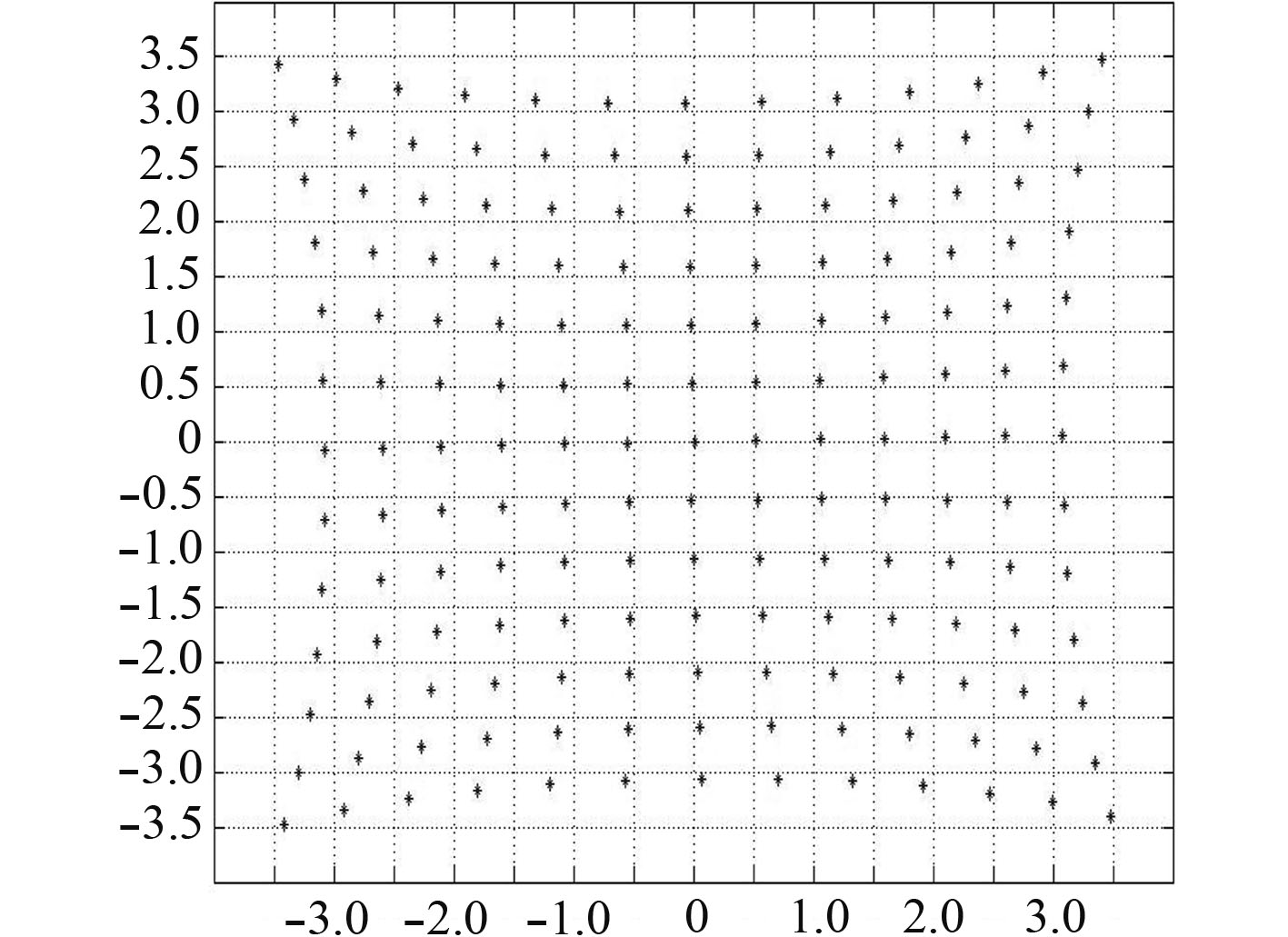图 4 原始实验数据点图 Fig. 4 Original experimental data point diagram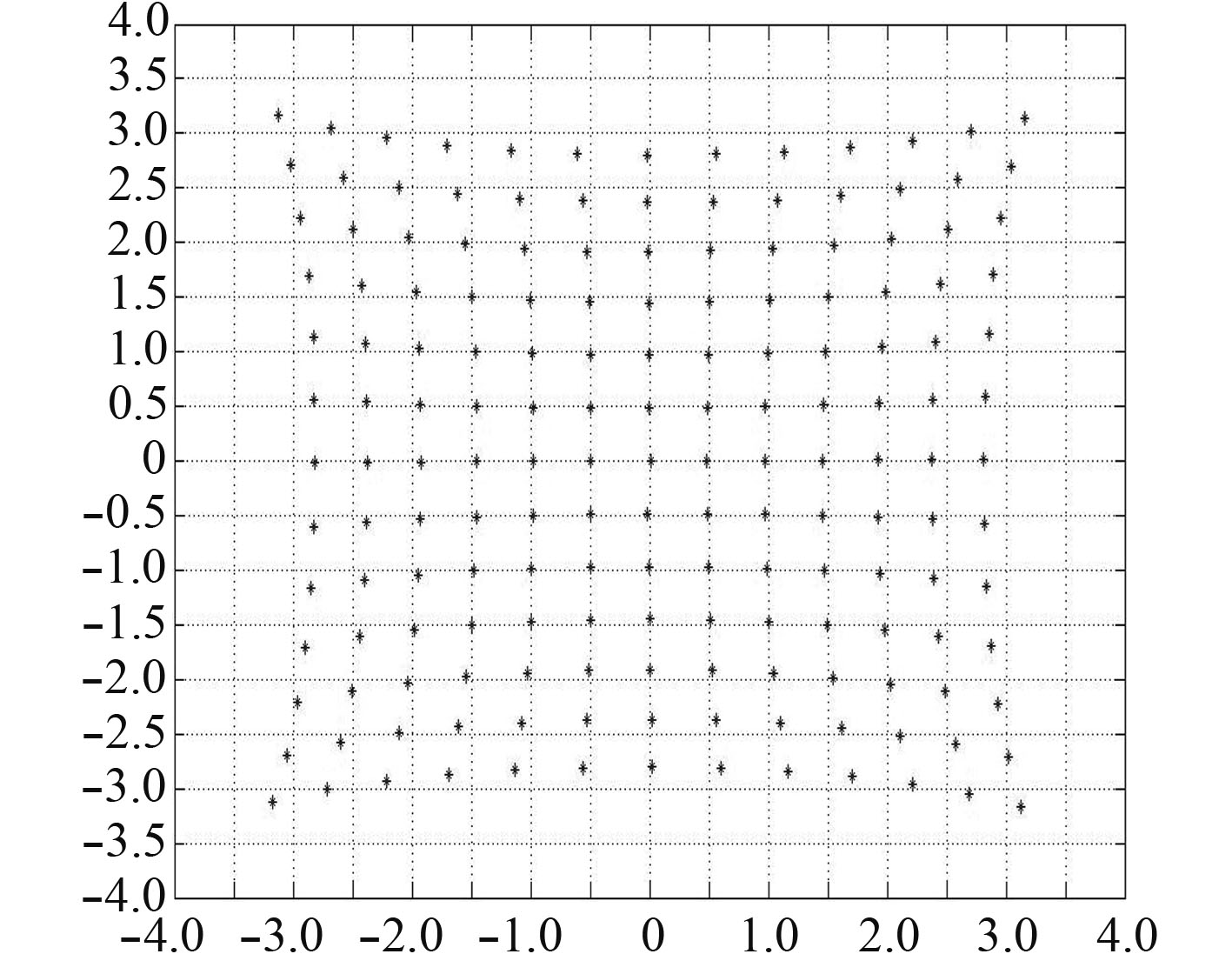图 5 二次多项式校正结果 Fig. 5 Quadratic polynomial correction result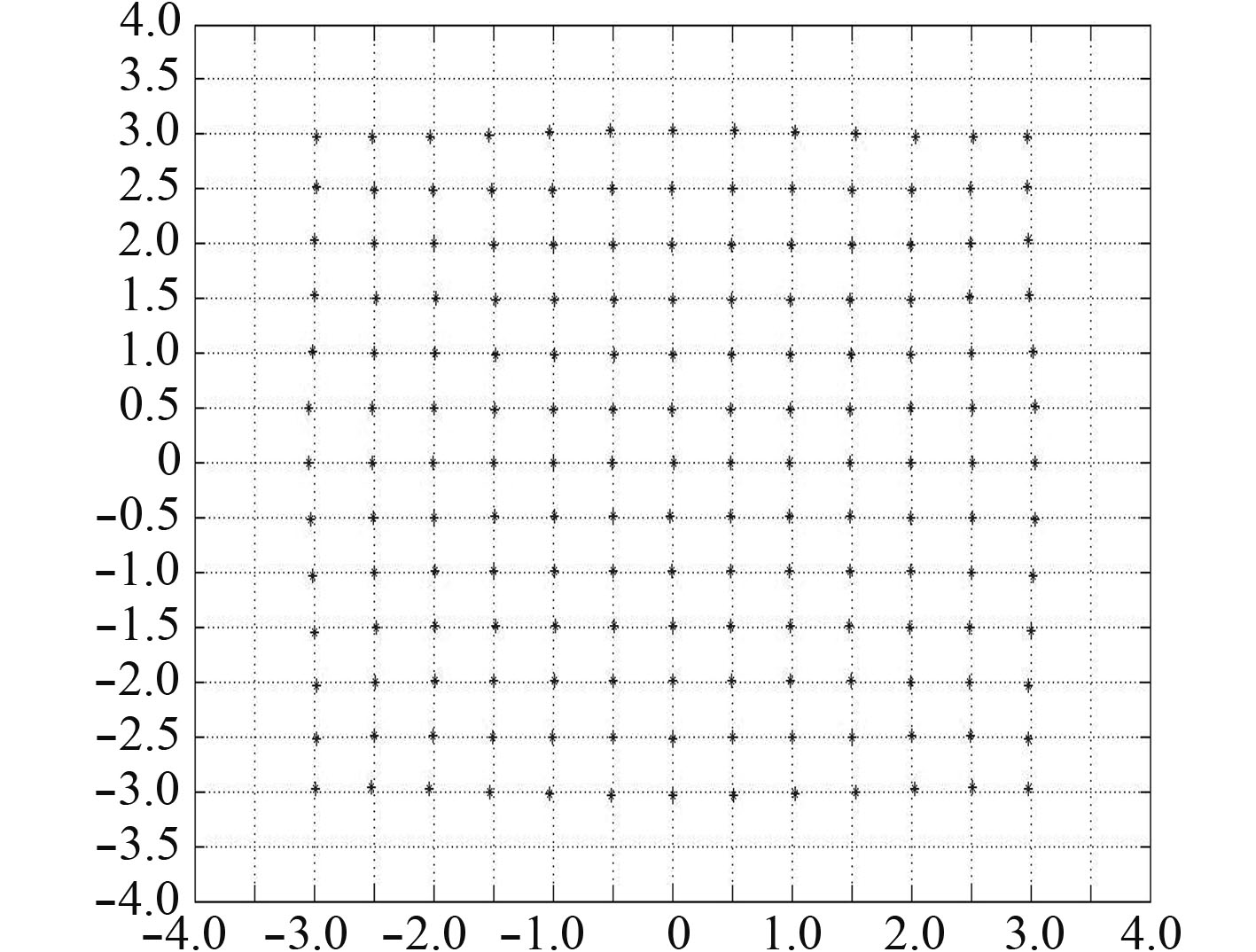图 6 三次多项式校正结果 Fig. 6 Cubic polynomial correction result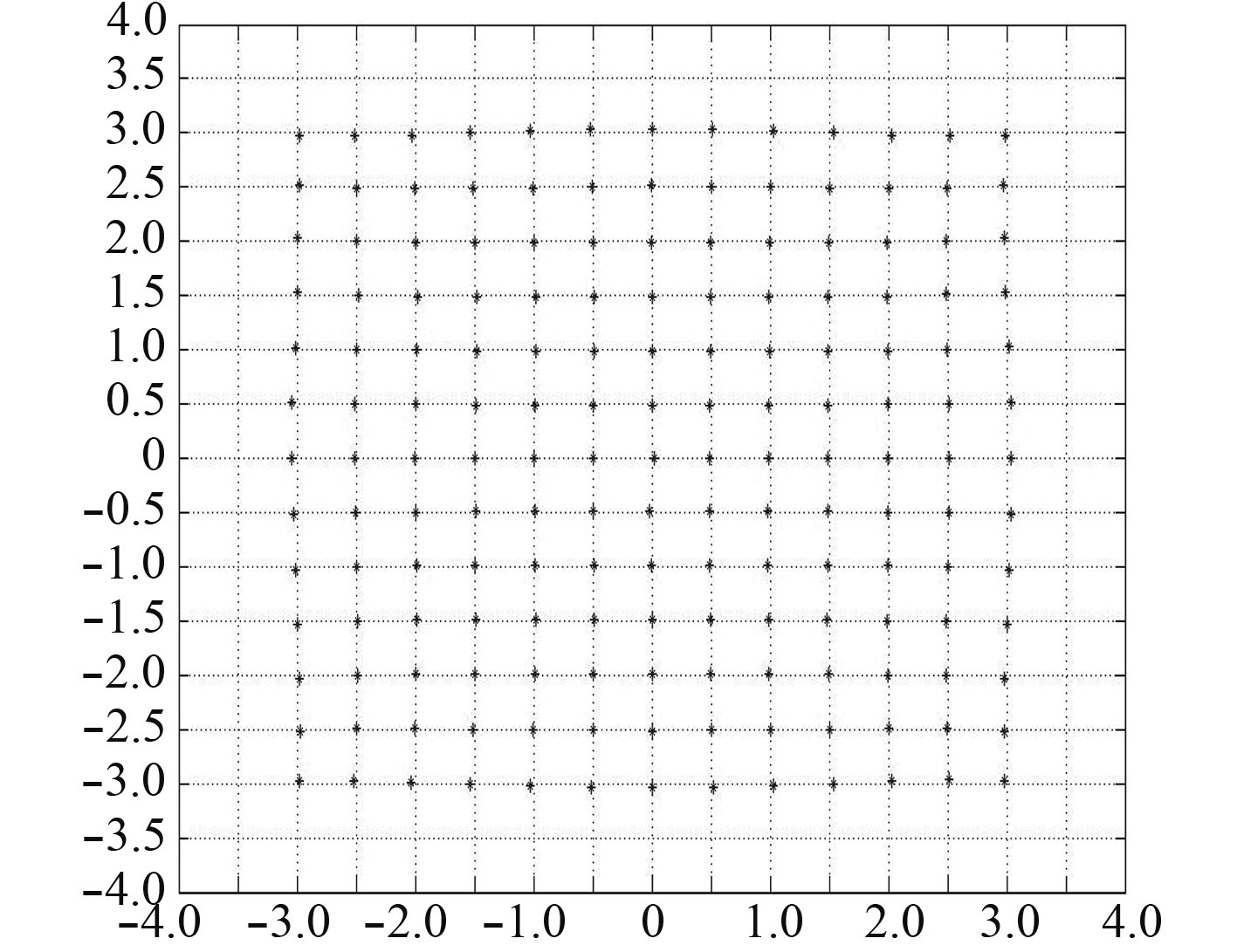图 7 四次多项式校正结果 Fig. 7 Quartic polynomial correction result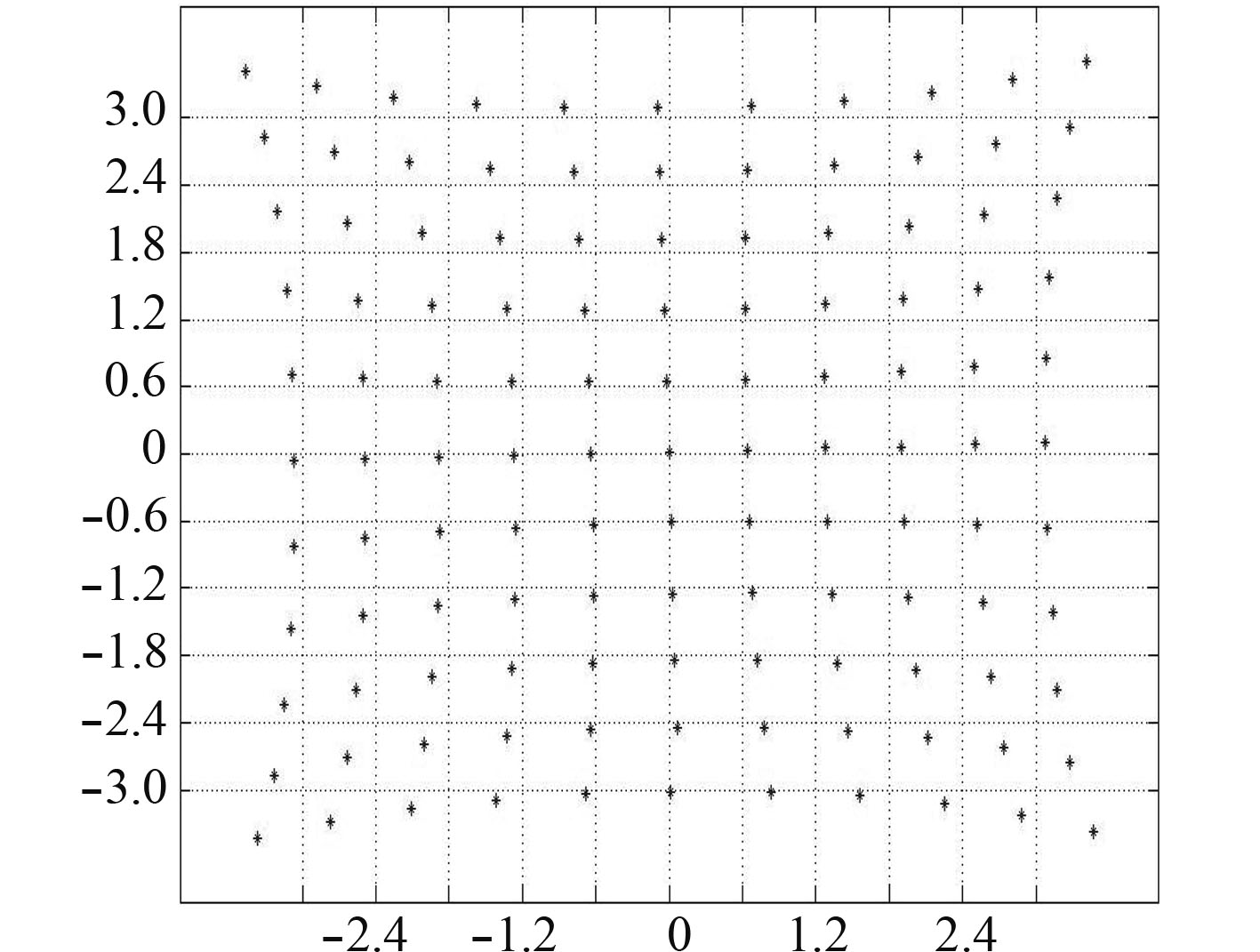图 8 原始数据点图 Fig. 8 Original data point diagram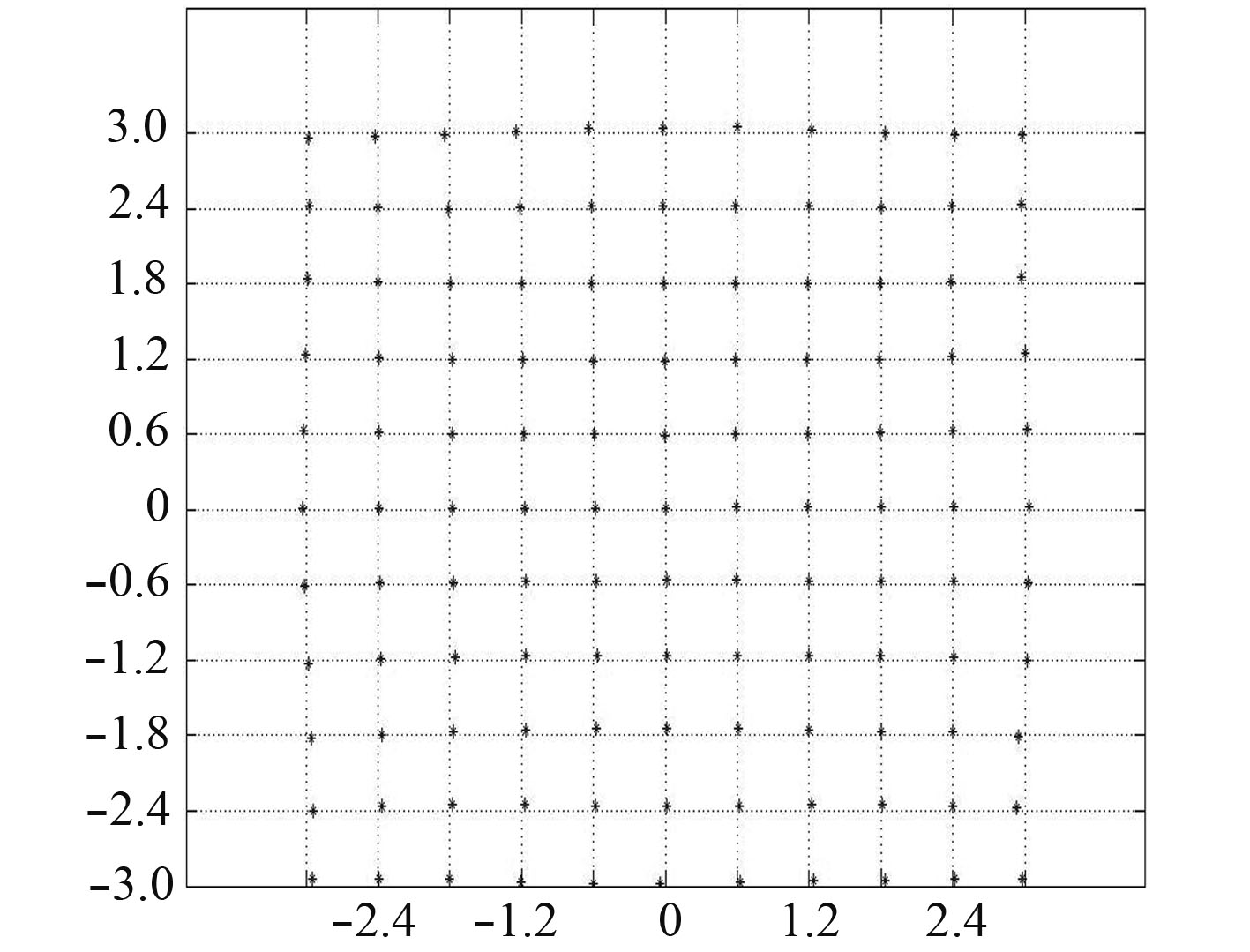图 9 校正后的结果 Fig. 9 Corrected result

  史春玉, 李田泽, 仝其丰, 等. 基于改进共轭梯度算法的二维PSD非线性修正[J]. 山东理工大学学报(自然科学版), 2017(2): 27-30. DOI:10.3969/j.issn.1672-6197.2017.02.006  周宝玉, 陈培锋, 朱明珠. 位置敏感探测器非线性误差修正实验研究[J]. 光学仪器, 2005(5): 13-17.  管炳良, 李星, 王俊. 二维PSD非线性修正技术研究[J]. 仪器仪表学报, 2007(7): 1295-1299. DOI:10.3321/j.issn:0254-3087.2007.07.027  谭超. 基于三次样条函数的传感器特性曲面二维插值[J]. 传感器技术, 2005(1): 69-71. DOI:10.3969/j.issn.1000-9787.2005.01.027  莫长涛, 陈长征, 张黎丽, 等. 二维PSD非线性修正的神经网络算法的研究[J]. 光电子·激光, 2003(4): 342-344. DOI:10.3321/j.issn:1005-0086.2003.04.003  张家田, 董秀莲. 非线性传感器的校正方法[J]. 石油工业技术监督, 2002(6): 27-29. DOI:10.3969/j.issn.1004-1346.2002.06.010  牟丽娜, 陈鸿, 陈闽鄂. 基于L-M算法的二维PSD非线性校正[J]. 计量与测试技术, 2006(12): 21-22. DOI:10.3969/j.issn.1004-6941.2006.12.013  徐士良. 数值分析与算法[M]. 北京: 机械工业出版社, 2003.266–276.